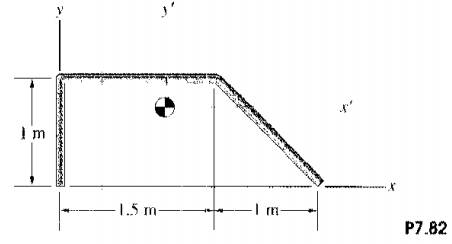### Create an Account

Home / Questions / Determine the mass moment of inertia of the bar in Problem 782 about the z axis through it...

# Determine the mass moment of inertia of the bar in Problem 782 about the z axis through its center or mass Problem 782 The mass of the homogeneous slender bar is 20 kg Determine its mass

Determine the mass moment of inertia of the bar in Problem 7.82 about the z’ axis through its center or mass.

Problem 7.82

The mass of the homogeneous, slender bar is 20 kg. Determine its mass moment of inertia about the z axis.Jun 15 2020 View more View LessSubscribe To Get Solution